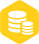# 单片机驱动发光二极管实现数码管时钟的设计

1760人已加入

### 描述

BeepP3^7

//作者：whw8099

#include

#include

#define uchar unsigned char

#define uintunsigned int

#defineKSET0x60 //设置当前时间键

#defineKSET_LONG0x61//设置闹铃时间键

#defineKINC0x50 //加1键

#defineKDEC0x30//减1键

sbitSPK=P3^7;//蜂鸣器控制引脚

uchar code MAX［2］={24，60};//时、分的最大值

uchar code segtab［］={0xc0，0xf9，0xa4，0xb0，0x99，0x92，0x82，0xf8，

0x80，0x90，0x88，0x83，0xc6，0xa1，0x86，0x8e，0x89，0x8c，0xff};

uchar dbuf［4］={0，0，0，0};//4位显示缓存

uintcount;//用于累加定时器T0的溢出中断次数，取值为0到4000

uchar time［3］={0，0，0}; //当前时、分、秒

uchar ala［2］={0，0};//报警时、分

ucharstate;//state=0：正常走时方式；

//state=1：设置方式；

//state=2：正在闹铃

bitupdate;//时间更改标志

uchar flash=00;//闪烁控制字

uchar disp_on=0xf0;//显示控制位

void delay（void）

{ uchar i;

for（i=0;i《200;i++）;

}

void hexbcd（uchar *tp）

{dbuf［3］=tp［0］/10;//将时拆分为BCD码送显示缓存

dbuf［2］=tp［0］|0x80;

dbuf［1］=tp［1］/10;//将分拆分为BCD码送显示缓存

dbuf［0］=tp［1］;

}

void disp（void）

{ static ucharcn=0;

uchar n，bsel;

bsel=0xfe;//最初点亮最低位

for（n=0;n《4;n++）

{ P2=bsel|disp_on;//位选口

P0=segtab［dbuf［n］&0x7f］;//显示缓冲单元的数据查出字段码表

if（（dbuf［n］&0x80）！=0）

P0=P0&0x7f;

bsel=_crol_（bsel，1）;//准备显示下一位

delay（）;//每位显示约1毫秒

P0=0xff;//熄灭数码管，防止当前位在下一位置显示出来

}

cn++;//每显示一遍，cn加1

if（cn==200）

{ disp_on=disp_on^flash;

dbuf［2］=dbuf［2］^0x80;

cn=0;

}

}

voidtime0（void） interrupt 1using 1

{ static uintn=0，m=0;

count++;//中断次数加1

if（count==4000）//满1s

{ count=0;//count清0

time［2］++;//秒加1

if（time［2］==60）//满60秒

{ time［2］=0;//秒清0

time［1］++;//分加1

if（state！=1） update=1; //更新标志

if（time［1］==60）//满60分

{ time［1］=0;//分清0

time［0］++;//时加1

if（time［0］==24）//满24小时

time［0］=0;//时清0

}

}

if（（state==0）&&（time［2］==0））//当前为走时状态且刚满1分（60秒）

{ if（（time［0］==ala［0］）&&（time［1］==ala［1］）） //闹铃时间到

{ flash=0x0f;//当前时间闪烁

disp_on=0xf0;

state=2;//当前状态设置为正在闹铃

dbuf［2］=dbuf［2］|0x80;//将时与分的分隔符显示出来

}

}

}

if（state==0x02）//如果当前正在闹铃

{ if（count%2==0）//如果满500？s（用来产生频率为1KHZ的方波）

{n++;

if（n《500）//在1秒的前1/4秒内

SPK=~SPK; //蜂鸣器发声

else

SPK=1;//产生3/4秒的停顿

if（n==2000）//如果满1 S（每次鸣叫间隔1S）

{n=0;//n清0，重新再由0计到2000（1S）

m++;//n计满2000（1S）m加1

if（m==6）//如果满6秒

{ state=0;//闹铃时间结束

m=0;//m清0

flash=0x00;//恢复正常显示

disp_on=0xf0;

}

}

}

}

}

uchar getkey（void）

{ ucharkey;

uchart;

disp（）;

if（（key=P1&0x70）==0x70） return 0xff;

for（t=0;t《5;t++） disp（）;

if（（key=P1&0x70）==0x70） return 0xff;

while（（P1&0x70）！=0x70）//检测按键时间

{ disp（）;

if（t《250） t++;

}

if（（t》200）&&（key==0x60）） return 0x61;

return key;

}

void set_time（uchar *tp）

{ uchar p=0;

uchar k;

state=1;//当前为设置状态

flash=0x0c;//LED显示器最高2位（时）闪烁

while（1）

{ hexbcd（tp）;//将要设置的时、分转换成BCD码送显示缓存

k=getkey（）;//读取按键键值

if（k==KSET）//如果是SET键

{ p++;//调整下标p，使其指向是时或分

p=p&0x01;//防止下标出界

disp_on=0xf0;//恢复正常显示

if（p==0x00） //如果指向的是时

flash=0x0c;//LED显示器的高2位闪烁

else

flash=0x03;//LED显示器的低2位闪烁

}

else if（k==KINC）//如果是加1键

{ tp［p］++;//时/分加1

if（tp［p］==MAX［p］） tp［p］=0;//检查时/分是否超出范围

}

else if（k==KDEC）//如果是减1键

{ tp［p］--;//时/分减1

if（tp［p］==0xff） tp［p］=MAX［p］-1;//检查时/分是否超出范围

}

else if（k==KSET_LONG）//如果长按SET键，准备返回

break;

}

hexbcd（time）;//恢复当前时间的显示

flash=0x00;//LED显示器恢复正常显示

disp_on=0xf0;

state=0;//当前状态恢复为正常走时状态

}

voidmain（void）

{

uchark;

state=0;//正常显示时钟方式

count=0;//计数器清0

flash=0x00;//显示器正常显示

disp_on=0xf0;

time［0］=12;//初始化当前时、分、秒

time［1］=25;

time［2］=55;

ala［0］=12;//初始化 时、分

ala［1］=26;

hexbcd（time）; //将当前时间转换为BCD码到显示缓存

TMOD=0x02; //T0工作于定时方式2

TH0=-250;//T0的定时时间为250？s

TL0=-250;

ET0=1;//允许T0中断

TR0=1;//启动定时

EA=1;//开中断

while（1）

{ disp（）;

k=getkey（）;//读取按键状态

if（state==0x02）//如果当前正在闹铃

{ if（k！=0xff）//只要有键按下

{ state=0x00; //退出闹铃方式，回到正常走时状态

flash=0x00; //正常显示，不闪烁

disp_on=0xf0;

}

}

else if （k==KSET_LONG）//如果长按SET键

{

set_time（ala）;//设置报警时间

}

else if（k==KSET）//如果是SET键

{ TR0=0;//关闭定时器T0

set_time（time）;//设置当前时间

TR0=1;//重新开启定时器

}

if（update==1）

{ update=0;

hexbcd（time）;

}

}

}×
20